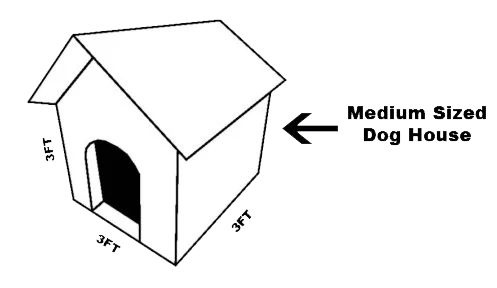# How To Calculate “Air Tank Fill Time” Using Simple Logic

May 2, 2023

If you’re wondering how to estimate the amount of time it will take to fill your air tank, you’ve come to the right place!

We’ll tell you what to do if you have an air tank or other suitably rated pressure vessel and want to figure out how long it will take to increase the pressure to a certain level using an air compressor with a known CFM capacity.

Best of all, we’ll calculate this time to fill an air tank using common sense and minimal formulas.

## 3 Things You Need To Know To Get Started

1. Atmospheric air at sea level has an Absolute Pressure of 14.7 psia
• Call it 15 psia for a rough estimate
• It is important to use psia when any pressure ratios are being considered
• psia is “psig (gauge) + 15 psi”
• Examples: If a pressure gauge is reading “0,” the absolute pressure is about 15 psia
• If a pressure gauge is reading “100,” the absolute pressure is about 115 psia
2. Air is essentially a perfect spring:3. The capacity of a compressor refers to the volume of atmospheric air that it can pull in and “process” (i.e., squeeze down or compress) in a given amount of time… And squeeze it down and discharge it along a pipe or hose at high pressure.

## How To Calculate Time To Fill An Air Tank Questions

With this background information in mind, we can calculate “time to fill an air tank” questions using simple logic. In the following examples, we demonstrate the logic one of VMAC’s air compressor engineers used to answer these questions so you can replicate similar logic during your own calculations.

### Example 1

How long will it take a 15 CFM compressor to fill a 100-gallon tank from 0 psig to 120 psig?

• 1 gallon is about 232 cubic inches
• 1 cubic foot is exactly 12 x 12 x 12 = 1728 cubic inches

#### Time To Fill Logic

1. 1 ft³ = 1728/232 = 7.5 gallons
2. The tank is 100/7.5 = about 13 ft³
3. Therefore, there is 13 ft³ of atmospheric air in the tank to start
4. There is 135/15 x 13 = 117 ft³ of atmospheric air packed into the tank when it is up to the target pressure of 120 psig (135 psia)
5. The difference is 117-13 = 104 ft³
6. The 15 CFM compressor processes 15 ft³ of atmospheric air every minute:
1. One minute produces 15 ft³
2. Two minutes produces 30 ft³
3. Four minutes produces 60 ft³
4. Seven minutes produces 105 ft³
7. Therefore, 104 ft³ / 15 ft³ per Min = 6.9 minutes.

It will take about 7 minutes.

### Example 2

How long will it take a 120 CFM compressor to take a 1-mile length of 6” schedule 40 pipe (deadheaded for testing) up to 30 psig from zero psig?

• Pipe ID is 6.07 inches
• The area of a circle is about ¾ the area of the square that surrounds it
• (6.07 x 6.07) x 0.75 = 27.6
• The pipe has an area of roughly 28 inches

#### Time To fill Logic

1. There are 144 square inches in one square foot (ft²)
1. Area = 28/144 = 0.19 ft²
2. The pipe volume is simply the circular area times length
1. Volume = 0.19 x 5280 ft (1 Mile) = 1000 ft³
3. There is 1000 ft³ of atmospheric air in the pipe to start
4. There will be 45/15 x 1000 = 3,000 ft³ of atmospheric air packed into the pipe when it is up to the target pressure of 30 psig
5. The difference is 3000 – 1000 = 2000 ft³
6. The 120 CFM compressor processes 120 ft³ of atmospheric air every minute:
1. One minute produces 120 ft³
2. Eight minutes produces 960 ft³
3. Sixteen minutes produces 1920 ft³
4. Seventeen minutes produces 2040 ft³
7. Therefore, 2000 ft³ / 120 ft³ per Min = 16.7 minutes

It will take about 17 minutes.

### Example 3

How long will it take a 30 CFM compressor to fill a 35 Gallon tank from 110 psig to 145 psig?

#### Time To Fill Logic

1. As we saw in Example 1, there are about 7 ½ gallons in a cubic foot. Therefore, 35 gallons is roughly 5 ft³
2. At 145 psig (160 psia), the tank will have 160/15 x 5 = 53 ft³ of atmospheric air in it
3. Likewise, at 110 psig, the tank will have 125/15 x 5 = 42 ft³ of atmospheric air in it
4. The difference is 11 ft³
5. A 30 CFM compressor processes 30 ft³ of atmospheric air every minute
• In 10 seconds, it produces 5 ft³ (1/6 of 30)
• In 20 seconds, it produces 10 ft³

It will take about 20 seconds to recover the tank to 145 psig.

With some basic knowledge of your compressor, air pressure and the amount of air you need, figuring out the time to fill can be relatively easy – without using complex formulas.

Want more? You may also like: